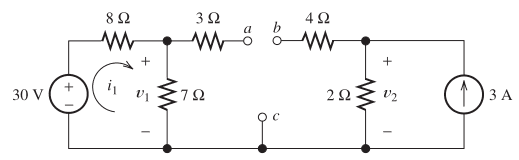# Basic Electric Circuit

• Engineering
• Graphite

## Homework Statement

Consider the circuit shown. Find the values of v1 and v2.## The Attempt at a Solution

I tried using KVL on the left closed loop and got:
$$v_{1} = 30 - 8i$$
I then used the current law between the two loops to get:
$$i_{1} - v_{1} / 7 = v_{2} / 2 - 3$$
That's where I'm stuck. Do the two resistors next to a and b have any current flowing through them? Would it also be possible to treat the 3A current source as a resistor and combine it with the ##2\Omega## resistor it's in parallel with? Thanks.

Perhaps try another approach. Are you familiar with the concept of a potential divider ?

If not then note that the current through the 3Ohm is zero and apply KCL to the node on the left of the 3Ohm. What does it tell you about the current through the 8 and 7 Ohm?

The current through the 4 Ohm is also zero.

Would it also be possible to treat the 3A current source as a resistor and combine it with the 2Ω 2\Omega resistor it's in parallel with?

Note the current through the 4Ohm is zero. Apply KCL to the node on the right of the 4Ohm. What does that say about the current through the 2 OHM?

Perhaps try another approach. Are you familiar with the concept of a potential divider ?

If not then note that the current through the 3Ohm is zero and apply KCL to the node on the left of the 3Ohm. What does it tell you about the current through the 8 and 7 Ohm?

The current through the 4 Ohm is also zero.
So the current for both the 8 ohm and 7 ohm resistor would be ##i_{1}## and the current through the 2 ohm resistor would be 3 A. I can see how to solve it now. I'm getting v1 = 14 and v2 = 6, which looks correct.

Thanks for the help.

Yes v1=14V and V2 =6V is correct.